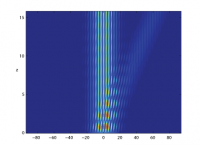## Use of Equivalent Hermitian Hamiltonian for PT-Symmetric Sinusoidal Optical Lattices

H. F. JonesWe show how the band structure and beam dynamics of non-Hermitian $PT$-symmetric sinusoidal optical lattices can be approached from the point of view of the equivalent Hermitian problem, obtained by an analytic continuation in the transverse spatial variable $x$. In this latter problem the eigenvalue equation reduces to the Mathieu equation, whose eigenfunctions and properties have been well studied. That being the case, the beam propagation, which parallels the time-development of the wave-function in quantum mechanics, can be calculated using the equivalent of the method of stationary states. We also discuss a model potential that interpolates between a sinusoidal and periodic square well potential, showing that some of the striking properties of the sinusoidal potential, in particular birefringence, become much less prominent as one goes away from the sinusoidal case.

http://arxiv.org/abs/1009.5784
Optics (physics.optics); Quantum Physics (quant-ph)